CBSE Class 10 Sample Paper for 2018 Boards

Class 10
Solutions of Sample Papers for Class 10 Boards

### An AP consists of 37 terms. The sum of the three middle most terms is 225 and the sum of the last three terms is 429. Find the AP.

This is a question of CBSE Sample Paper - Class 10 - 2017/18.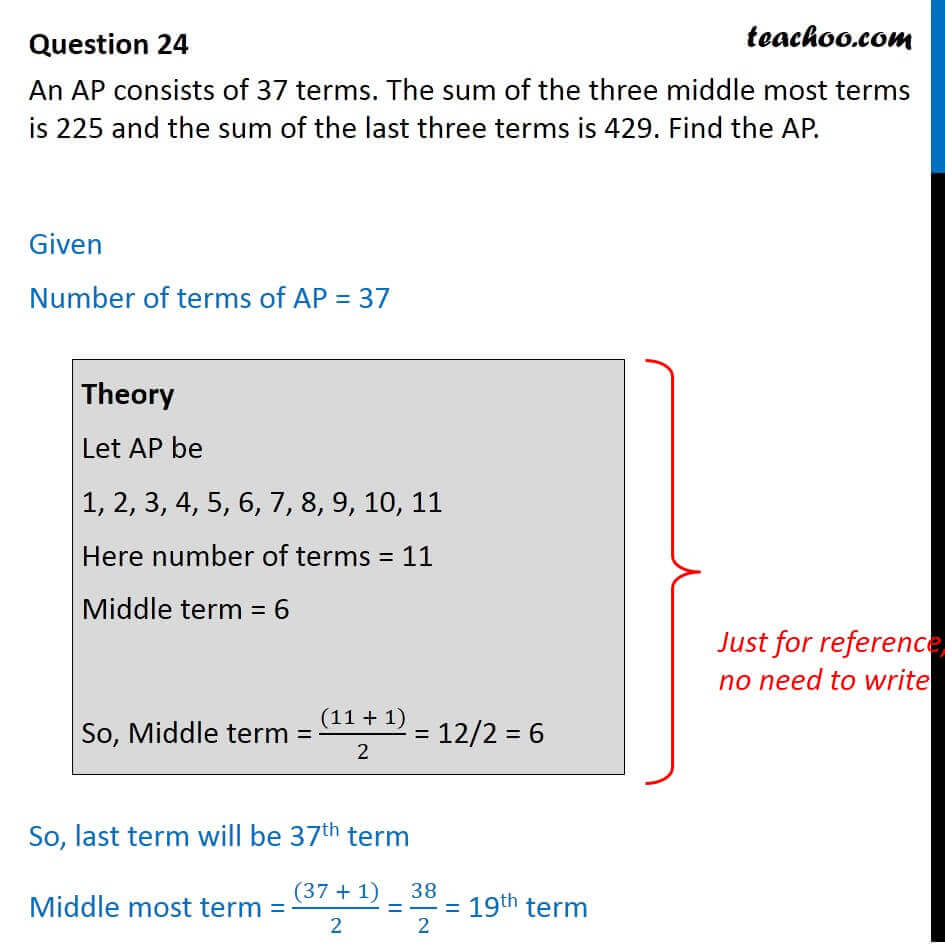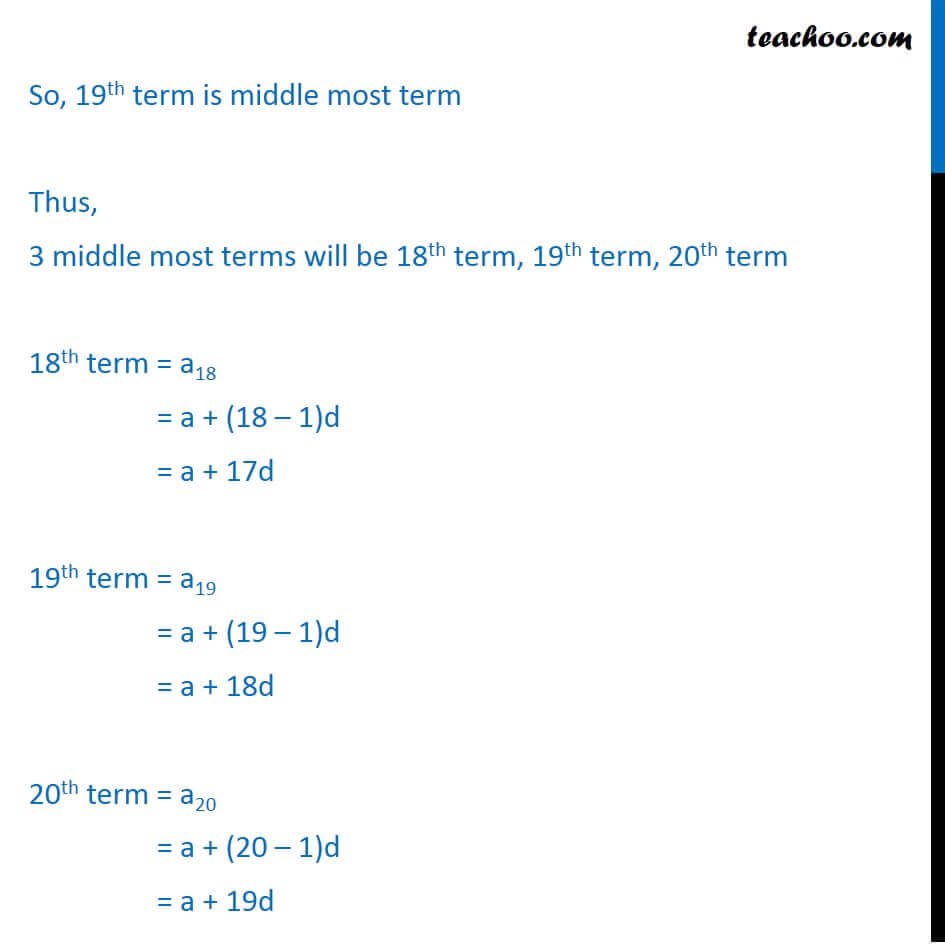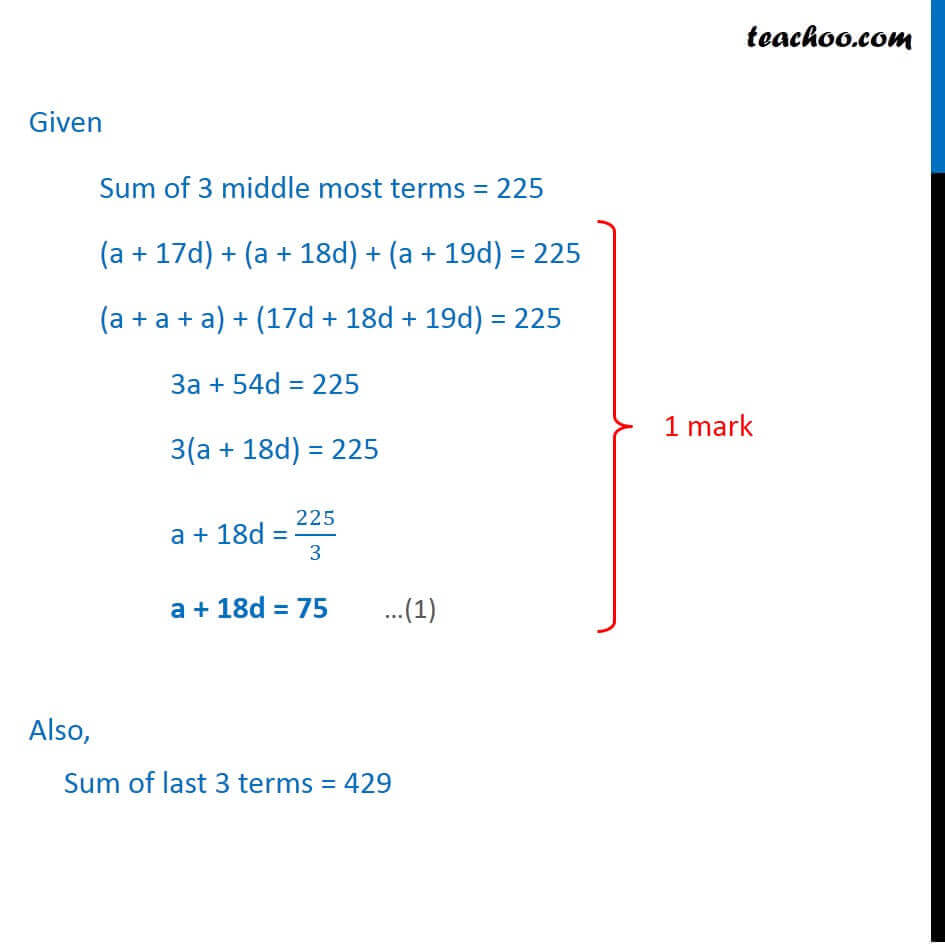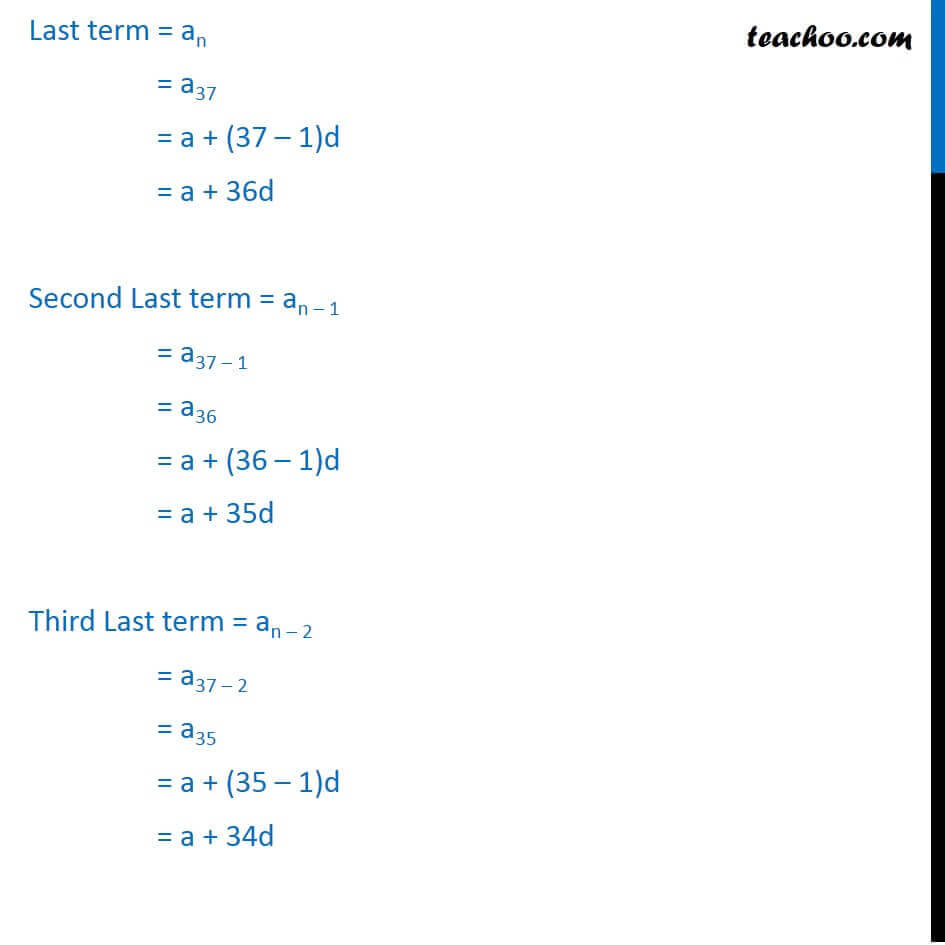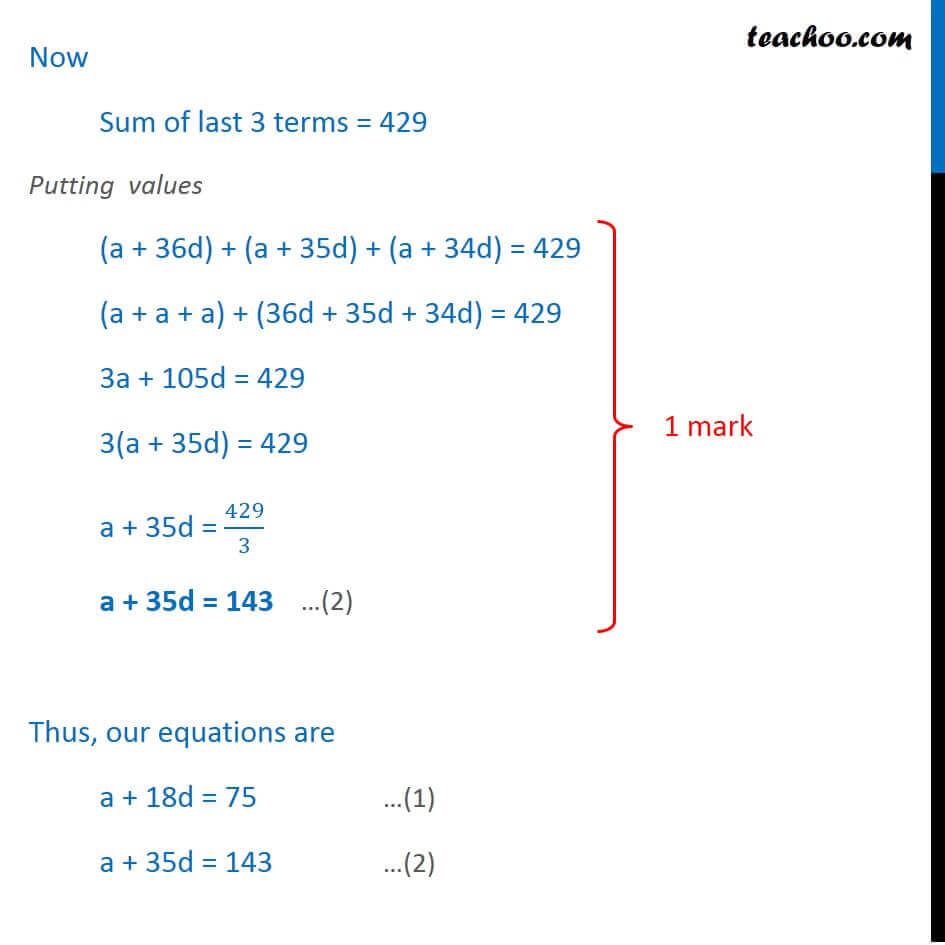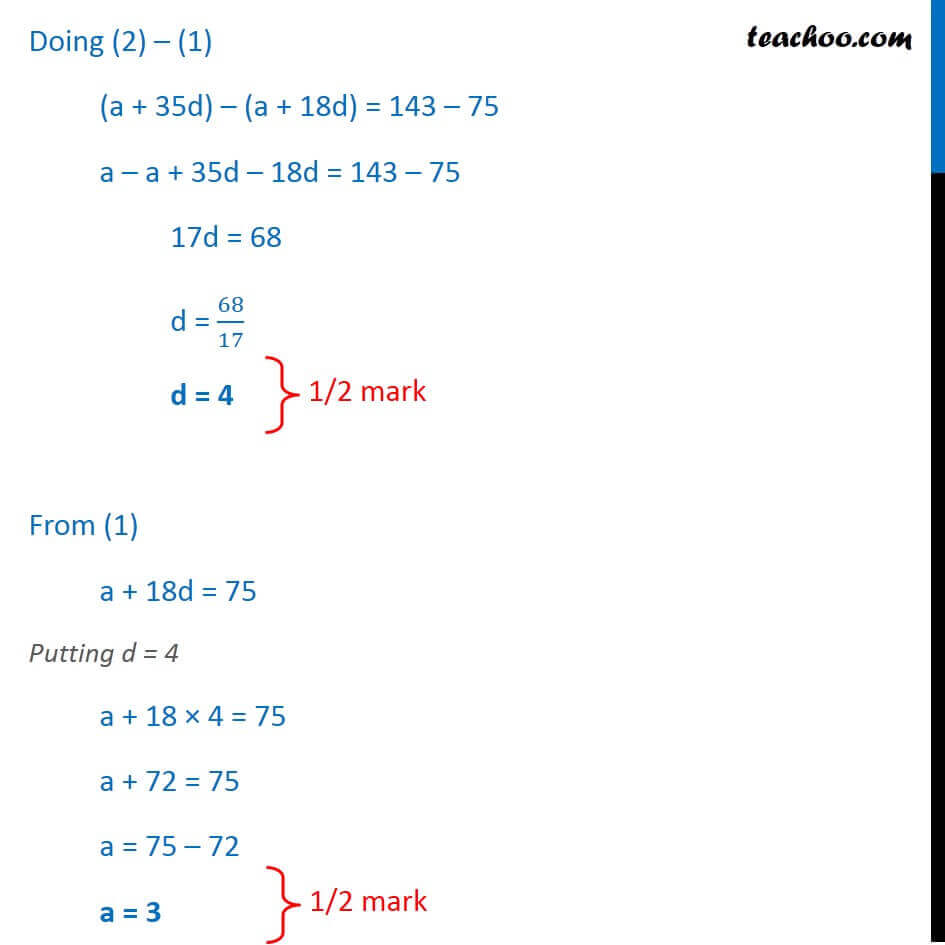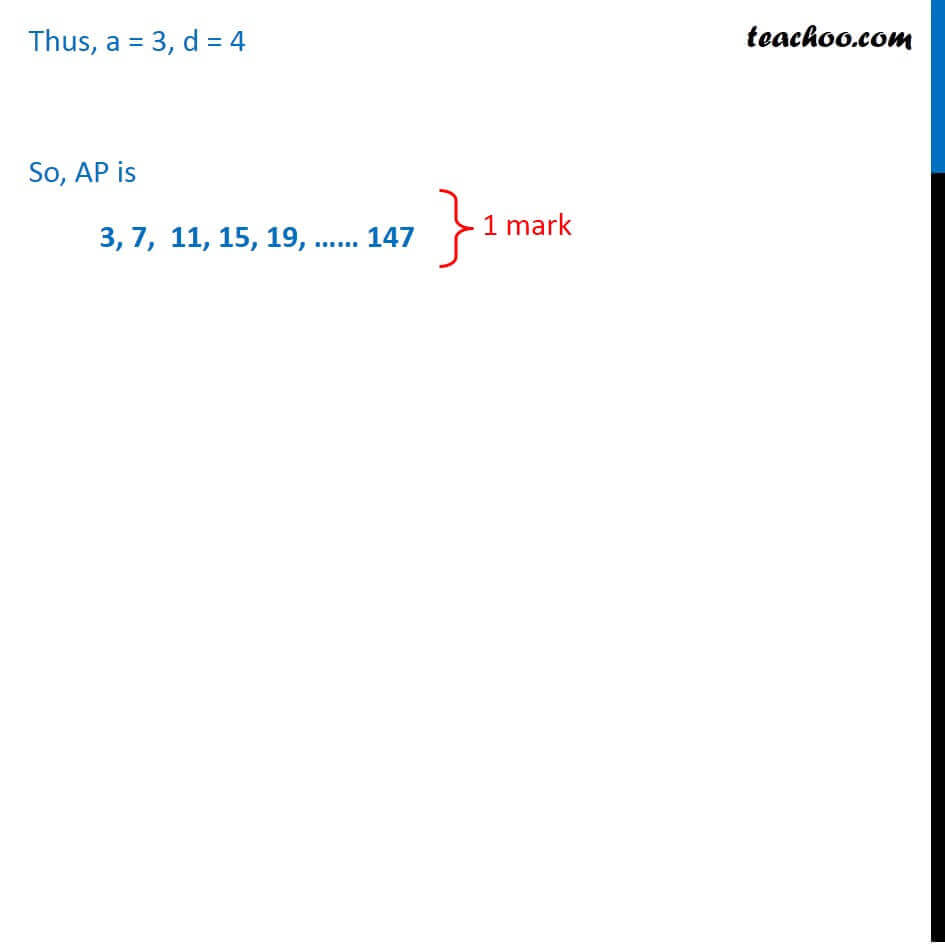Learn in your speed, with individual attention - Teachoo Maths 1-on-1 Class

### Transcript

Question 24 An AP consists of 37 terms. The sum of the three middle most terms is 225 and the sum of the last three terms is 429. Find the AP. Given Number of terms of AP = 37 So, last term will be 37th term Middle most term = ((37 + 1))/2 = 38/2 = 19th term Theory Let AP be 1, 2, 3, 4, 5, 6, 7, 8, 9, 10, 11 Here number of terms = 11 Middle term = 6 So, Middle term = ((11 + 1))/2 = 12/2 = 6 So, 19th term is middle most term Thus, 3 middle most terms will be 18th term, 19th term, 20th term 18th term = a18 = a + (18 – 1)d = a + 17d 19th term = a19 = a + (19 – 1)d = a + 18d 20th term = a20 = a + (20 – 1)d = a + 19d Given Sum of 3 middle most terms = 225 (a + 17d) + (a + 18d) + (a + 19d) = 225 (a + a + a) + (17d + 18d + 19d) = 225 3a + 54d = 225 3(a + 18d) = 225 a + 18d = 225/3 a + 18d = 75 Also, Sum of last 3 terms = 429 Last term = an = a37 = a + (37 – 1)d = a + 36d Second Last term = an – 1 = a37 – 1 = a36 = a + (36 – 1)d = a + 35d Third Last term = an – 2 = a37 – 2 = a35 = a + (35 – 1)d = a + 34d Now Sum of last 3 terms = 429 Putting values (a + 36d) + (a + 35d) + (a + 34d) = 429 (a + a + a) + (36d + 35d + 34d) = 429 3a + 105d = 429 3(a + 35d) = 429 a + 35d = 429/3 a + 35d = 143 Thus, our equations are a + 18d = 7 5 …(1) a + 35d = 143 …(2) Doing (2) – (1) (a + 35d) – (a + 18d) = 143 – 75 a – a + 35d – 18d = 143 – 75 17d = 68 d = 68/17 d = 4 From (1) a + 18d = 7 5 Putting d = 4 a + 18 × 4 = 75 a + 72 = 75 a = 75 – 72 a = 3 Thus, a = 3, d = 4 So, AP is 3, 7, 11, 15, 19, …… 147